Technical Information Site of Power Supply Design

2015.11.12 DC/DC

# Operating Principles of Buck Switching Regulator

Switching Regulator Basics

Whereas in the preceding section we explained that the switching regulator is capable of performing buck (step down), boost (step up), buck/boost (step down and up), and inverting conversions, moving forward we describe the operating principles of the switching regulator, taking the buck converter, which is a most frequently used DC/DC converter, as an example.

Figure 31 is a schematic view of DC/DC buck conversion that converts DC voltage denoted VIN by time-division switching and soothing the output by means of an inductor and capacitor to produce the desired DC voltage.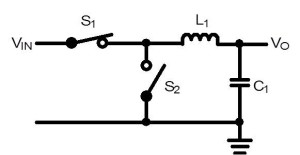Figure 31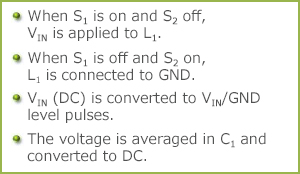Figure 32

In a nutshell, the DC/DC conversion process involves the conversion of DC to AC, smoothing it, and reconverting it to DC. In terms of a PWM operation as an example, if with S1=ON/S2=OFF the time to supply VIN is set to 25%, and if with S1=OFF/S2=ON the zero-voltage state (GND) is set to a 75% pulse cycle and the pulses are averaged, a 25% DC is produced. If VIN is 10V, Vo will be 25%, or 2.5V. For ease of visualization, the process may be depicted in terms of areas (see Figure 33).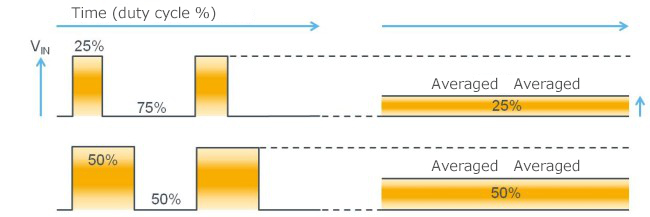Figure 33

In actual PWM, since the load current of the averaged output fluctuates, if the ON time remains constant the voltage ends up increasing or decreasing as a function of the load current, which defeats the purpose of constructing a regulator. Therefore, when the output declines, the ON time is increased, and more energy is supplied from the input side to increase the output voltage. When the output voltage has been restored sufficiently, the ON time is shortened to suppress the rise of the output.

In the case of switching regulator, the process may be thought of as drawing the power necessary for the output from the input, as may be clear from the figure. By contrast, because the linear regulator does not turn on/off as switching, it operates in a 100% duty cycle, that is, the input is never turned off. By the same token, if 10V is converted to 2.5V, the power equivalent to the 7.5V difference is wasted in the form of heat. It is this difference in the mechanism of operations that accounts for the high efficiency of the switching regulator.

## Keypoint

・The switching regulator delivers high efficiency by chopping (switching) the input DC voltage and transmitting only the required power to the output side.

Nonsynchronous (diode) rectifying buck converter : Its circuitry and operation
Figure 34 is a modification of Figure 31 to make it a little more specific. This circuit is referred to as a nonsynchronous or diode rectifying DC/DC conversion. In this circuit S1 represents a switch (normally a transistor) and S2 has been replaced by a diode which operates in a like manner. The red line indicates the current path when S1 is on, and the green line, when S1 is off.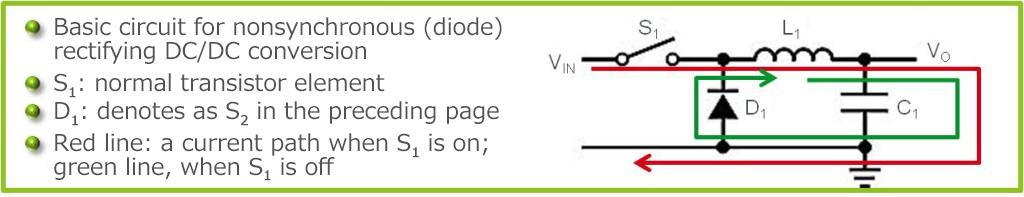Figure 34

Figure 35 illustrates voltages and current waveforms in each part. In the previous section it was stated that “DC/DC conversion process converts DC to AC, and smooths out the output.” If you wonder if the input is converted to “AC (alternating current in which the amplitude and the positive and negative directions change periodically)”, you may be able to tell that the output is AC by looking at the inductor voltage waveform.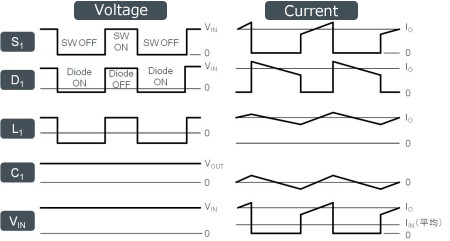Figure 35

Incidentally, the reason that the inductor current waveform is tilted is that because the rage of change in the inductor current moves in proportion to the voltage, and when a voltage is applied, the current increases at a fixed slope, which can be expressed by the equation V=L×(dI/dt).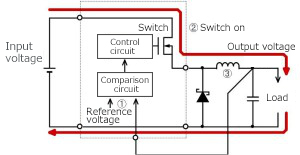Figure 36. Specific nonsynchronous rectifying buck circuit and current path with the switch ON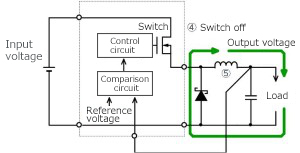Figure 37. Current path when the switch is off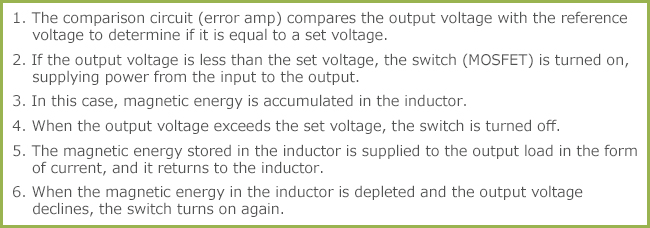Figure 38

Figures 36 and 37 represent a substitution of the circuit in Figure 35 for the actual circuit. The switch S1 is replaced with a MOSFET, and S2 with a Schottky diode. The comparison and control circuits, omitted in Figure 35, are also shown in the new figures. As you may have noticed, this circuit is identical to the feedback control circuit that was explained in connection with the operating principles of the linear regulator. The output voltage, pulled into an internal comparison circuit, is compared with the reference voltage. The term “comparison circuit” used here is synonymous with the error amp used in the linear regulator. Whereas in the linear regulator the output from the error amp directly controlled the output transistor and continuously performed loop controls, in the switching regulator the output from the error amp is replaced by control of the on/off time (duty cycle) for the switch (transistor).

#### Key Points:

・In a DC/DC conversion, DC is switched (the energy required for output is divided by time) and converted to AC (in which the inductor voltage swings between positive and negative), and the output is rectified and smoothed for reconversion to DC.

・The roles of the switch and inductor should be understood from the flow of currents. The debugging process requires a solid understanding of the waveform for each node.

This website uses cookies.

By continuing to browse this website without changing your web-browser cookie settings, you are agreeing to our use of cookies.